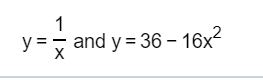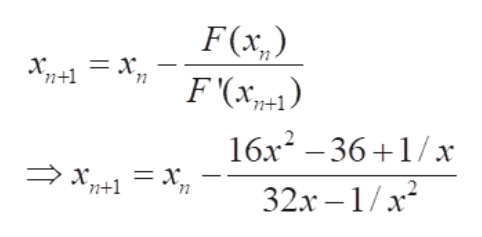1yand y 36 16xX

Question

Use Newton's method to approximate all intersection points of the following pair of curves.

Round to six decimal places as needed.help_outlineImage Transcriptionclose1 yand y 36 16x X fullscreen
Step 1

Before we get into the solution of the question, let's understand a bit about Newton's method.

If xn is an approximate solution of F(x) and F’(xn) ≠ 0 then the next approximate solution, xn+1  is given by the expression on the White Board.

Step 2
Step 3

Based on the pair of curves given to us, let's define F(x) as:

F(x) = 1/x – (36 – 16x2)  = 16x2 - 36 + 1 /x

Hence, F'(x) = 32...help_outlineImage TranscriptioncloseF(x,) F(x п+1 71 16х? — 36 +1/х п+1 72 32х —1/x2 fullscreen

Want to see the full answer?

See Solution

Want to see this answer and more?

Our solutions are written by experts, many with advanced degrees, and available 24/7

See Solution
Tagged in

Derivative